# The triangles

The triangles ABC and A'B'C 'are similar with a similarity coefficient of 2. The angles of the triangle ABC are alpha = 35°, beta = 48°. Determine the magnitudes of all angles of triangle A'B'C '.

A2 =  35 °
B2 =  48 °
C2 =  97 °

### Step-by-step explanation:

${B}_{2}=\beta =48=48\text{°}$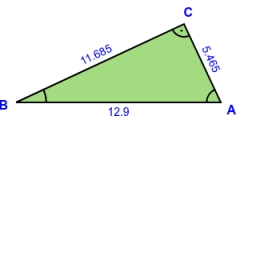Did you find an error or inaccuracy? Feel free to write us. Thank you!Tips to related online calculators

#### You need to know the following knowledge to solve this word math problem:

We encourage you to watch this tutorial video on this math problem:

## Related math problems and questions:

• Similarity coefficient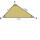The triangles ABC and A "B" C "are similar to the similarity coefficient 2. The sizes of the angles of the triangle ABC are α = 35° and β = 48°. Find the magnitudes of all angles of triangle A "B" C ".
• Two anglesThe triangles ABC and A'B'C 'are similar. In the ABC triangle, the two angles are 25° and 65°. Explain why in the triangle A'B'C 'is the sum of two angles of 90 degrees.
• TrianglesFind out whether given sizes of the angles can be interior angles of a triangle: a) 23°10',84°30',72°20' b) 90°,41°33',48°37' c) 14°51',90°,75°49' d) 58°58',59°59',60°3'
• Angles of a triangleIn triangle ABC, the angle beta is 15° greater than the angle alpha. The remaining angle is 30° greater than the sum of the angles alpha and beta. Calculate the angles of a triangle.
• Find theFind the third interior angle of the triangle ABC where: α = 48°, γ = 65°.
• SimilarityAre two right triangles similar to each other if the first one has an acute angle 70°, and the second one has an acute angle 20°?
• MO Z7–I–6 2021In the triangle ABC, point D lies on the AC side and point E on the BC side. The sizes of the angles ABD, BAE, CAE and CBD are 30°, 60°, 20° and 30°, respectively. Find the size of the AED angle.
• Area and two anglesCalculate the size of all sides and internal angles of a triangle ABC, if it is given by area S = 501.9; and two internal angles α = 15°28' and β = 45°.
• Gamma angleFind the magnitude of the gamma angle in triangle ABC if: α = 38° 56 ’and β = 47° 54’.
• The two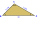The two angles of a triangle are 78° and 82°. So what is the measure of the remaining third angle?
• AircraftFrom the aircraft flying at an altitude of 500m, they observed places A and B (located at the same altitude) in the direction of flight at depth angles alpha = 48° and beta = 35°. What is the distance between places A and B?
• 3-bracket 3Two angles in a triangle are 90° and 60°. Has triangle at least two equal sides?
• Angles of the triangleABC is a triangle. The size of the angles alpha, beta are in a ratio 4: 7. The angle gamma is greater than the angle alpha by a quarter of a straight angle. Determine angles of the triangle ABC.
• The triangles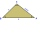The triangles KLM and ABC are given, which are similar to each other. Calculate the lengths of the remaining sides of the triangle KLM, if the lengths of the sides are a = 7 b = 5.6 c = 4.9 k = 5
• Similarity coefficientIn the triangle TMA the length of the sides is t = 5cm, m = 3.5cm, a = 6.2cm. Another similar triangle has side lengths of 6.65 cm, 11.78 cm, 9.5 cm. Determine the similarity coefficient of these triangles and assign similar sides to each other.
• The angles ratioThe angles in the ABC triangle are in the ratio 1: 2: 3. find the sizes of the angles and determine what kind of a triangle it is.
• Angles in triangle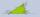Calculate the alpha angle in the triangle if beta is 61 degrees and 98 gamma degrees.